### IMO Shortlist 1978 problem 4

Kvaliteta:
Avg: 0,0
Težina:
Avg: 0,0
Let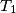$T_1$ be a triangle having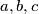$a, b, c$ as lengths of its sides and let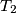$T_2$ be another triangle having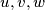$u, v,w$ as lengths of its sides. If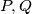$P,Q$ are the areas of the two triangles, prove that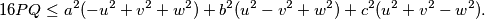When does equality hold?
Izvor: Međunarodna matematička olimpijada, shortlist 1978